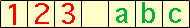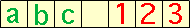Subject: Frogs and lily pads Name: Nick Who is asking: Student Level: Secondary Question: There are 5 lily pads and 4 frogs, 2 Red and 2 Green, how many moves does it take for them to swap sides? Answer: 8 I have a formula which will tell you how many moves it will take with different total numbers of frogs, it is [(F*F)/4]+F Where F is the number of red frogs add the number of Green frogs. This formula only works with numbers of frogs that are the same on each side. What I would like to know is why this formula works and why you have to divide it by 4? Please help! Nick Hi Nick, I assume that the rules for moving are that a frog can move to the next lily pad if it is vacant or he can jump over a frog to the vacant lily pad. Suppose that there are h red frogs and h green frogs. I want to first look at the situation where the each frog keeps its position relative to the other frogs of the same colour. So, for example if h = 3 and the red frogs are 1, 2, and 3 and the green frogs are a, b and c in the starting positionsthen the ending positions will beIn this situation each of the 2h frogs has moved h + 1 positions and thus there have been 2h(h + 1) = 2h2 + 2h position changes. Each move is either a small jump to an adjacent lily pad or a large jump over another frog to a lily pad two steps away. If S is the number of small jumps and L is the number of large jumps then the total number of position changes is S + 2L. Thus S + 2L = 2h2 + 2h. But the number of moves is S + L and hence the number of moves is S + L = 2h2 + 2h - L Since each frog keeps its position relative to the other frogs of the same colour it only has to jump over the h frogs of the other colour. Thus each frog makes h large jumps and thus L = h2. Thus the total number of moves is S + L = 2h2 + 2h - L = 2h2 + 2h - h2 = h2 + 2h This is exactly your expression since F = 2h. The way the question is worded - how many moves does it take for them to swap sides? - implies the minimum number of moves is required. If you looked at any arrangement of the end position other than the one discussed, provided you never make backward moves, you will end up with the same total number of position moves. To see this consider first the simple case in which two of the frogs have 'swapped' positions (they are not really swapping positions, we just view the final result as if they had). One has travelled farther than in the case we have discussed while the other has travelled less, by exactly the same amount. Thus the solution would be the same. We now need to observe that any final permutation of the frogs, on either of the sides, can be achieved by a sequence of k such 'swaps' of two frogs. We may consider an appropriate permutation achieved by k-1 'swaps'. Our previous argument indicates the total number of position moves to get this end point is 2h2 + h. By our previous argument the permutation with k swaps can be done in the same number of position moves. Cheers, Penny Go to Math Central